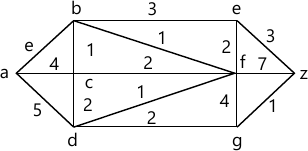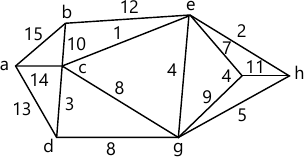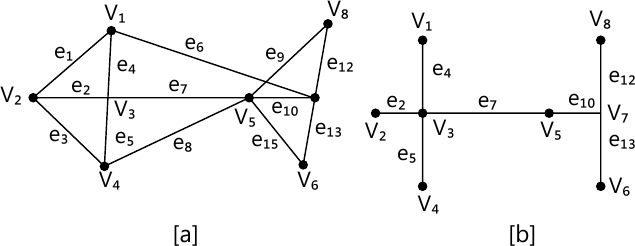MORE IN Discrete Mathematics
SPPU Computer Engineering (Semester 3)
Discrete Mathematics
June 2015
Total marks: --
Total time: --
INSTRUCTIONS
(1) Assume appropriate data and state your reasons
(2) Marks are given to the right of every question
(3) Draw neat diagrams wherever necessary

Answer any one question from Q1 and Q2
1 (a) In the first year computer science class of 80 students, 50 knew COBOL, 55 'C' and 46 PASCAL. It was also known that 37 knew 'C' and COBOL, 28 'C' and PASCAL, and 25 PASCAL and COBOL. 7 students however knew none of the language.
(i) How many knew all the three languages?
(ii) How many knew exactly two languages?
(iii) How many knew exactly one language?
4 M
1 (b) Let n be a positive integer Sn, be the set of all divisors of n. Let D denote the relation of 'division'. Draw the diagrams of lattices for:
(i) n = 24
(ii) n = 30
(iii) n = 6.
4 M
1 (c) Negative each of the statement:
i) ∀x, |x|=x
ii) &exists;x, x2=x
iii) If there is a riot, them someone is killed.
iv) It is day light all the people are arisen.
4 M

2 (a) Find the transitive closure of the relation R on:
A = {1, 2, 3, 4) defined by
R = {(1, 2), (1, 3), (1, 4), (2, 1), (2, 3), (3, 4), (3, 2), (4, 2), (4, 3)}
4 M
2 (b) Prove by mathematical induction that for : n > = 1:
1.1!+2.2!+3.3!+....+n.n!=(n+1)!=1.
4 M
2 (c) Let f(x) = x + 2, g(x) = x ? 2 h(x) = 3x for x R where R is the set of real number,
find:
(i) gof (ii) fog (iii) fof (iv) hog
(v) gog (vi) foh (vii) hof (viii) fohog.
4 M

Answer any one question from Q3 and Q4
3 (a) Show that in a connected planar graph with 6 vertices and 12 edges, each of the regions is bounded by 3 edges:
5 M
3 (b) Show that (G/N, *) is a group.
4 M
3 (c) Explain the terms:
(i) Homomorphism of Group
(ii) Automorphism of Group.
4 M

4 (a) Find the shortest path between a-z for the given graph : using Dijkstra's algorithm.6 M
4 (b) Show that R = {a + b; b l) for the operation +, * is an integral domain but not a field.
5 M
4 (c) Explain the term Eulerian path and Eulerian circuit with Example
2 M

Answer any one question from Q5 and Q6
5 (a) Obtain the minimum spanning tree using Kruskal's algorithm for the following graph. Obtain the total cost of minimum spanning tree.7 M
5 (b) Find the fundamental system of cut-set for the graph G shown below with respect to the spanning tree T.5 M

6 (a) A secondary storage media contains information in files with different formats. The frequency of different types of files is as follows: exe (20), bin(75), bat(20), jpeg(85), dat(51), doc(32), sys(26), c(19), cpp(25), bmp(30), avi(24), prj (29), 1st(35), zip(37).
Construct the Huffman code of this.
6 M
6 (b) Explain:
(i) Eccentricity of a vertex
(ii) Cut points
(iii) Level and height of a tree.
6 M

Answer any one question from Q7 and Q8
7 (a) Suppose license plate contains 3 English letters followed by 4 digits:
i) How many different license plates can be manufactured if repetition of letters and digits are allowed?
(ii) How many plates are possible if only the letters are Repeated?
(iii) How many plates are possible if only the digits are repeated ?
7 M
7 (b) Out of 4 officers and 10 clerks, a committee of 2 officers and 3 clerks is to be formed. In how many ways committee can be done if:
(i) Any officer and any clerk can be included.
(ii) A particular clerk must be in committee.
(iii) A particular officer cannot be in committee.
6 M

8 (a) A student is to answer 10 out of 13 questions in an exam:
(i) How many choices has he, if he must answer the first or second questions but not both?
(ii) How many choices has he, if he must answer exactly three out of first five questions.
6 M
8 (b) Three students A, B and C are swimming in the race. A and B have, same probability of winning and each is twice as likely to win as C. Find the probability that:
(i) B wins
(ii) C wins
(iii) B or C wins.
7 M

More question papers from Discrete Mathematics